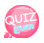# while statement

The while statement is a loop statement.
Loop statement can continue running while a value satisfies that condition.
For example, if you need to print 1 screen 200 times, you can use the loop to print 200 times instead of typing 1 200 times.
Usage is as follows.

```while(condition){
Statement to run while the condition is satisfied
}```

For example, if you make a while statement that outputs 1 to 10, it is as follows:

```<!DOCTYPE html>
<html>
<meta charset="utf-8" />
<title>JavaScript</title>
<script>
a = 1;
while(a <= 10){
document.write(a);
a++;
}
</script>
<body>
</body>
</html>```

In the source above, after declaring the value of a as 1, the condition is that a is less than or equal to 10. In the meantime, there is a statement that outputs the value of a and a ++ which raises the value of a by 1.
If you don't have a ++ in the source above, you will end up with an infinite loop since there is no ability for a to increase to 10. Please test it when you run the above sources.
Here is the source to find the cumulative sum of 1 to 10: Please figure out the algorithm. ^^ There are some places where this simple problem is surprisingly surprising.

```<!DOCTYPE html>
<html>
<meta charset="utf-8" />
<title>JavaScript</title>
<script>
a = 1;
sum = 0;
while(a <= 10){
sum += a;
document.write('Accumulated sum'+sum+"<br />");
a++;
}
</script>
<body>
</body>
</html>```

This time, let's learn about do ~ while statements.

### do ~ while statement

In the while statement above, statements are executed if the condition is true.
Do ~ while statement executes once and then executes the statement if it is true and exits.
Let's take a look at the structure.

### do ~ while statement structure

do{
condition is true, the command to execute is written here.
}
while(write a conditional expression here.)

Let's try to understand what do ~ while statements are through the source.

```    a = 1;
do{
document.write(a);
}
while(a==10)```

Looking at the source above, a = 1.
The conditional expression is 1 == 10, which means that we run the iterations while a is equal to 10.
In other words, 1 == 10 is not the same in the conditional expression, so we don't execute the loop. However, once the execution statement in the do statement is executed and the conditional expression is checked, it is executed once.
In other words, if a value of 1 is output once, the conditional expression is not checked and the statement is not executed in the do statement.
Then test it by watching the whole source. ^^

```<!DOCTYPE html>
<html>
<title> david's Web Laboratory</title>
<style type="text/css">
</style>
<script type="text/javascript">
a = 1;
do{
document.write(a);
a++;
}
while(a==10)
</script>